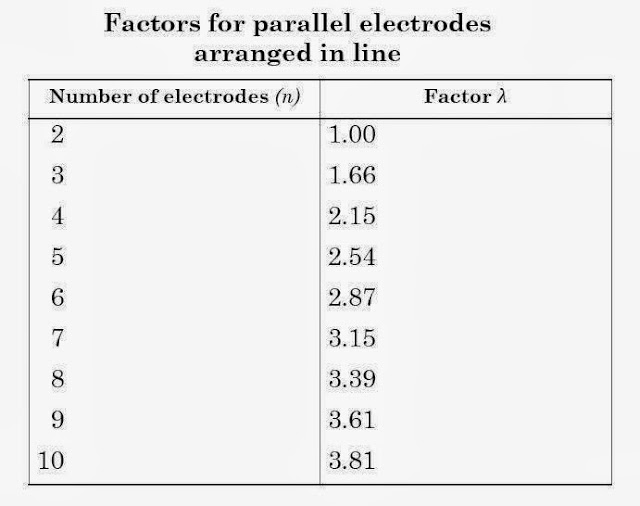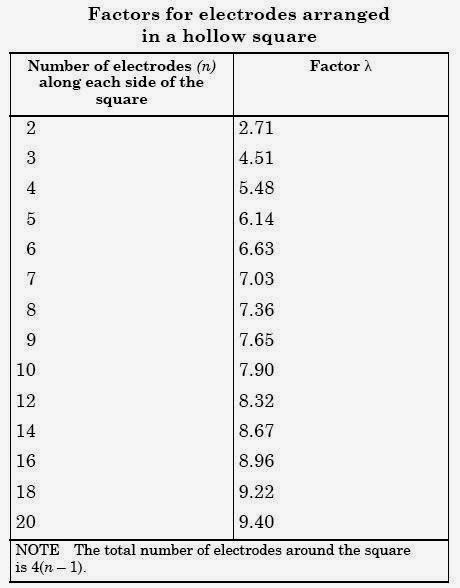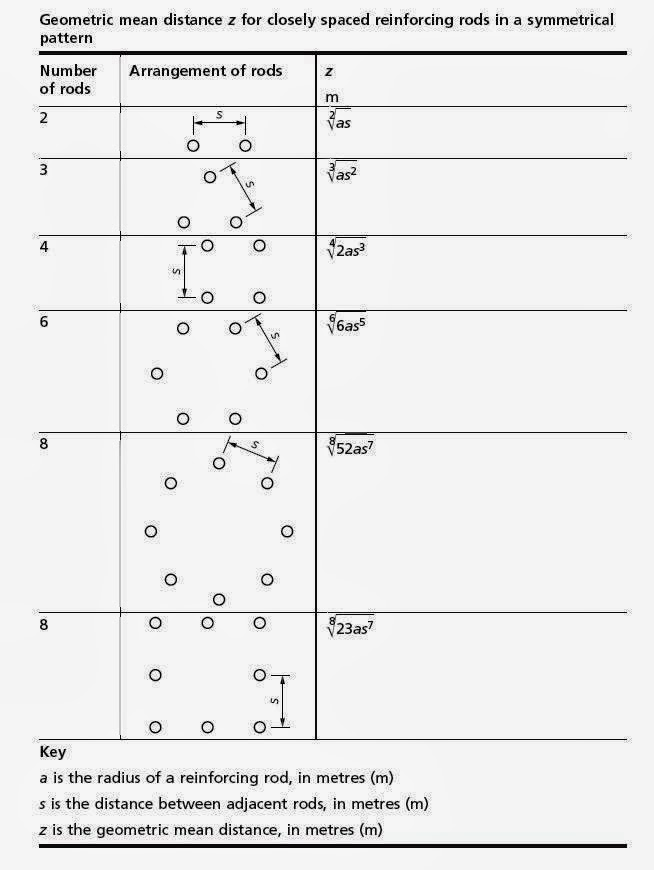### Grounding Design Calculations – Part Two

I indicated that the Earthing Systems Design Steps process has (3) main steps:

 Earthing Systems Design Steps A grounding system design process has (3) main steps: Data Collection, Data Analysis, Grounding Design Calculations.

And I explained the first step: Data Collection in the following Articles:

I explained the second step: Data Analysis in the following Articles:

And I explained What we are going to design for grounding system in any building in the following Articles:

And, in Article " Grounding Design Calculations – Part One ", I indicated the following:

 Grounding System Design Calculations according to type of the building The procedures for performing the Grounding System Design Calculations can differ slightly according to the type of the building as follows: Domestic, commercial and industrial premises, High and medium voltage electricity substations.

 First: Domestic, commercial and industrial premises We mean by domestic, commercial and industrial premises, all installations up to 1,000 V ac and 1,500 V dc - between phases, with some minor exceptions.

 Methods of Grounding Design Calculations There are many methods can be used for performing Grounding System Design Calculations But the common methods are: Equations Method, Nomographs Method, Excel Spreadsheets Method, Tables Method, Online Earthing Calculators Method, Software Programs Method.

In this Article, I explained the first method of grounding design calculations: Equations Method.

Today, we will solve some examples using the Equations Method but from BS 7430 – issued in 1998.

Examples for Grounding System Design Calculations by using the Equations Method from BS 7430 – issued in 1998.

 Some Rules/Equations from BS 7430: 1998 Revision

1- Parallel Rod Electrodes

The combined resistance of n rod electrodes in parallel can be obtained from the following equations:

Where:

Rn is the resistance of n rods in parallel, in ohms
R is the resistance of one rod in isolation, in ohms
n is the number of parallel rods
S is the distance between the adjacent rods, in meters
ρ is the resistivity of the soil, ohm-m

λ is the constant factor dependent on geometric arrangement of the electrodes and can be obtained from:

• Table-1: if the rods are equally spaced in a straight line.
• Table-2: if the rods are equally spaced around a hollow square, e.g. around the perimeter of a building.
• In case of three rods placed in an equilateral triangle, λ = 1.66.Table-1Table-2

Note to table 2:

Table 2 may also be used for electrodes arranged in a rectangle, where n is given by ( 1 + total number of electrodes/4) provided that the length to width ratio of the rectangle does not exceed 2, the error will be less than -6%.

Notes to parallel rods equation:

The above equation of parallel rods assumes the following:

• The rod electrodes can be represented approximately by hemispherical electrodes, having the same earthing resistance, located in the soil surface.
• The spacing between the rods is not less than their length.

 Example#1: For three rods placed in equilateral triangle, calculate the resistance to earth noting that: Length of the copper clad steel rod (L) = 3.0 m Diameter of the copper clad steel rod (D) = 16mm=0.016 m The resistivity of the soil (ρ) = 20.0 ohm-m 3 rods will be driven into the ground at a separation distance (S) of 3m. Solution: For three rods placed in equilateral triangle, λ = 1.66 (From BS7430:1998) Step#1: Calculate The Resistance Of One Rod Therefore: R = 6.70 ohms Step#2: Calculate The Resistance Of The (3) Parallel Rods    Where, R = 6.70 ohms; λ = 1.66; n = 3 So, Rn = 2.02 ohms.

2- Horizontal strip or round conductor electrodes

• Horizontal strip or round conductor electrodes have special advantages where high resistivity soil underlies shallow surface layers of low resistivity.
• They are frequently in the form of untinned copper strip of not less than 25 mm by 3 mm section, but may be of bare copper conductor as used for overhead lines.

For a strip or round conductor electrode the resistance R, in ohms is given by the following equation:

Where:

L is the length of the strip or conductor, in metres (m);
h is the depth of electrode, in metres (m);
w is the width of strip or diameter of conductor, in metres (m);
ρ is the resistivity of soil, in ohm metres (7·m);
P and Q are coefficients given in Table-3 for different arrangements of electrode.Table-3

Example#2:

For a building, 17m width and 35m length, Calculate the earth resistance by using the following electrode types:
1. Vertical copper bonded steel rod with length 3.0m, diameter 15mm and distributed as shown in Fig.1.
2. Copper lattice plate 600 mm x 600 mm and 1.5 mm thickness as shown in Fig.2

Noting that The measured sand soil resistivity ρ = 70.3 Ω.m.

Solution:

Case#1: Design Using Vertical Copper Bonded Steel Rod as in Fig.1

The resistance of one vertical rod calculated as follow:

So,

R=   70.3 * { loge (8*3 / 0.015) -1 } /2*3.14*3 = 23.79 ohm

The combined resistance of rod obtained from the following equation:

Where:

R: Resistance of one rod in isolation in ohm,
S: Distance between adjacent rods, in m
ρ: Resistivity of soil, Ωm,
λ: Factor depend on number of electrode,
n: Number of electrodes = 4
α= 70.3/2 π*23.79*5 = 0.094,
Rn = 23.79 {(1+1*0.094)/2}=7.46 ohm by use 4 pits

Take into account the resistance of cable connecting all rods of cross section area 120mm2 cu:

Where:

R = Resistance in ohms,
ρ = Soil resistivity in ohm metres (Ω-m),
L = Length of electrode in metres,
w = Width of strip or diameter of circular electrode in metres,
h = Depth of electrode in metres,
P and Q = Coefficients for different arrangements of electrode which given in table-2

L : Length of cable = 25m,
h : Depth of cable = 0.5m,
w : Diameter of cable 120mm2 cu = 0.017m

The combined earth resistance of the 4 rods and cable will be as follows:

It's recommended to bonding the earth pits to the concrete foundation of building, and the earth resistance of reinforcing column calculated by the following equation:

Where:

R = Resistance in ohms,
ρ = Soil resistivity in ohm metres (Ω-m),
ρc = Concrete resistivity in ohm metres (Ω-m),
L = Length of reinforcing rod below ground level in metres,
δ= thickness of concrete between rods and soil in meters,
Z = geometric mean distance of rod cluster in meters from Table-4,Table-4

From Table-4, we find Z equal to:

a: radius of reinforcing rod, s: distance between reinforcing rods (s = 0.03m, a = 0.008m so Z= 0.045m)

so, R= 14.38 ohm

The combination resistance of earth copper rods & cable with reinforcing concrete electrode: R1= 1.99 Ohm, R2 = 14.38 Ohm

Case#2: Design Using Copper Lattice Plate as in Fig.2

The resistance to earth of a plate electrode is given by the following equation:

Where:

R = Resistance in ohms,
ρ = Soil resistivity in ohm metres (Ω-m),
A = The Area of one face of the plate, in m2.

By using 4 buried plates: R1=R2=R3=R4=36.7 Ohm

The combination resistance of earth copper plats and the resistance of cable connecting all plates

The combination resistance of earth copper plate & cable and reinforcing concrete electrode

at the end,for this example, we find that:

Ground Resistance in Case#1: Using Vertical Copper Bonded Steel Rod = 1.74 ohm Ground Resistance in Case#2: Design Using Copper Lattice Plate = 1.82 ohm.

To download a Solved example for Grounding Design Calculations of small substation using Equations method from BS 7430-2011 Revision, please click on the link.

In the next Article, I will explain Other Methods of Grounding Design Calculations. Please, keep following.

#### 1 comment:

1.Hello there,
how to resgiater in this SITE